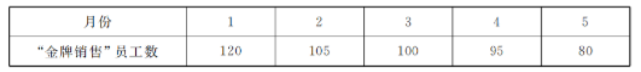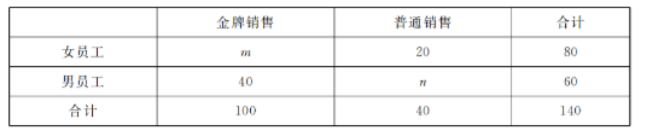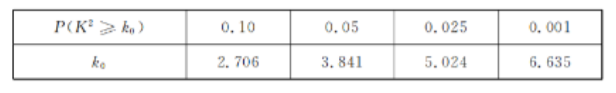(1) 由表中看出, 可用线性回归模型拟合“金牌销售” 员丁数 $y$ 与月份 $x$ 之间的关系, 求 $y$ 关于 $x$ 的 回归直线方程 $y=b x+a$, 并预测该房地产公司 6 月份犾得 “金牌销售” 称号的员工人数;
(2) 为了进一步了解员工们的销售情况, 选取了员工们在 3 月份的销售数据进行分析, 统计结果如下:\begin{aligned} K^2= & \frac{n(a d-b c)^2}{(a+b)(c+d)(a+c)(b+d)} \\ & (\text { 其中 } n=a+b+c+d) . \end{aligned}